This document contains R versions of the boxed examples from Chapter 9 of the “Analysis and Interpretation of Freshwater Fisheries Data” book. Some sections build on descriptions from previous sections, so each section may not stand completely on its own. More thorough discussions of the following items are available in linked vignettes:

The following additional packages are required to complete all of the examples (with the required functions noted as a comment and also noted in the specific examples below).

> library(FSA)           # Summarize, fitPlot, addSigLetters, residPlot
> library(NCStats)       # chisqPostHoc
> library(lattice)       # bwplot, xyplot
> library(multcomp)      # glht, mcp
> library(nlme)          # lme
> library(pgirmess)      # kruskalmc
> library(TeachingDemos) # chisq.detail

In addition, external tab-delimited text files are used to hold the data required for each example. These data are loaded into R in each example with read.table(). Before using read.table() the working directory of R must be set to where these files are located on your computer. The working directory for all data files on my computer is set with

> setwd("C:/aaaWork/Web/fishR/BookVignettes/aiffd2007")

In addition, I prefer to not show significance stars for hypothesis test output, reduce the margins on plots, alter the axis label positions, and reduce the axis tick length. In addition, contrasts are set in such a manner as to force R output to match SAS output for linear model summaries. All of these options are set with

> options(width=90,continue=" ",show.signif.stars=FALSE,
contrasts=c("contr.sum","contr.poly"))
> par(mar=c(3.5,3.5,1,1),mgp=c(2.1,0.4,0),tcl=-0.2)

## 9.1 Testing for Differences in Mean Length by Means of Analysis of Variance (ANOVA)

### 9.1.1 Preparing Data

The Box9_1.txt data file is read and the structure is observed below.

> d1 <- read.table("data/Box9_1.txt",header=TRUE)
> str(d1)
'data.frame':   360 obs. of  2 variables:
$lake : Factor w/ 3 levels "Island","Mitchell",..: 1 1 1 1 1 1 1 1 1 1 ...$ length: int  122 126 129 130 130 132 132 135 135 136 ...

### 9.1.2 ANOVA Results

The one-way ANOVA model is fit in R with lm() where the first argument is a formula of the form response~factor and the data= argument set equal to the data frame containing the variables. The ANOVA table with type I SS is then extracted from the lm object with anova().

> lm1 <- lm(length~lake,data=d1)
> anova(lm1)
           Df Sum Sq Mean Sq F value    Pr(>F)
lake        2  49324 24662.1   81.73 < 2.2e-16
Residuals 357 107725   301.7
Total     359 157049                          

### 9.1.3 Multiple Comparisons – Tukey Method

The Tukey multiple comparisons results are obtained by submitting the lm object as the first argument to glht(), from the multcomp package. This function requires a second argument that indicates which type of multiple comparison procedure to use. This second argument uses mcp() which requires the factor variable set equal to the word “Tukey” to perform the Tukey multiple comparison procedure. The saved glht object is submitted to summary() to get the difference in means with a corresponding hypothesis test p-value among each pair of groups and to confint() to get the corresponding confidence intervals for the difference in means. In addition, submitting the saved glht object to cld() will produce “significance letters” to indicate which means are different (different letters mean different means).

> mc1 <- glht(lm1,mcp(lake="Tukey"))
> summary(mc1)
                         Estimate Std. Error t value Pr(>|t|)
Mitchell - Island == 0     28.315      2.371  11.944   <1e-04
Thompson - Island == 0      6.105      2.232   2.735   0.0179
Thompson - Mitchell == 0  -22.210      2.191 -10.138   <1e-04
> confint(mc1)
                         Estimate lwr      upr
Mitchell - Island == 0    28.3151  22.7375  33.8926
Thompson - Island == 0     6.1052   0.8536  11.3569
Thompson - Mitchell == 0 -22.2098 -27.3642 -17.0555
> cld(mc1)
  Island Mitchell Thompson
"a"      "c"      "b" 

A graphic of the model results is obtained with fitPlot(), from the FSA package, and the significance letters are placed on the means plot with addSigLetters(). (addSigLetters() is from the NCStats package. You should examine the help for this function to see what each of the arguments is used for).

> fitPlot(lm1,ylab="Total Length (mm)",xlab="Lake",main="")
> addSigLetters(lm1,lets=c("c","a","b"),pos=c(2,2,4))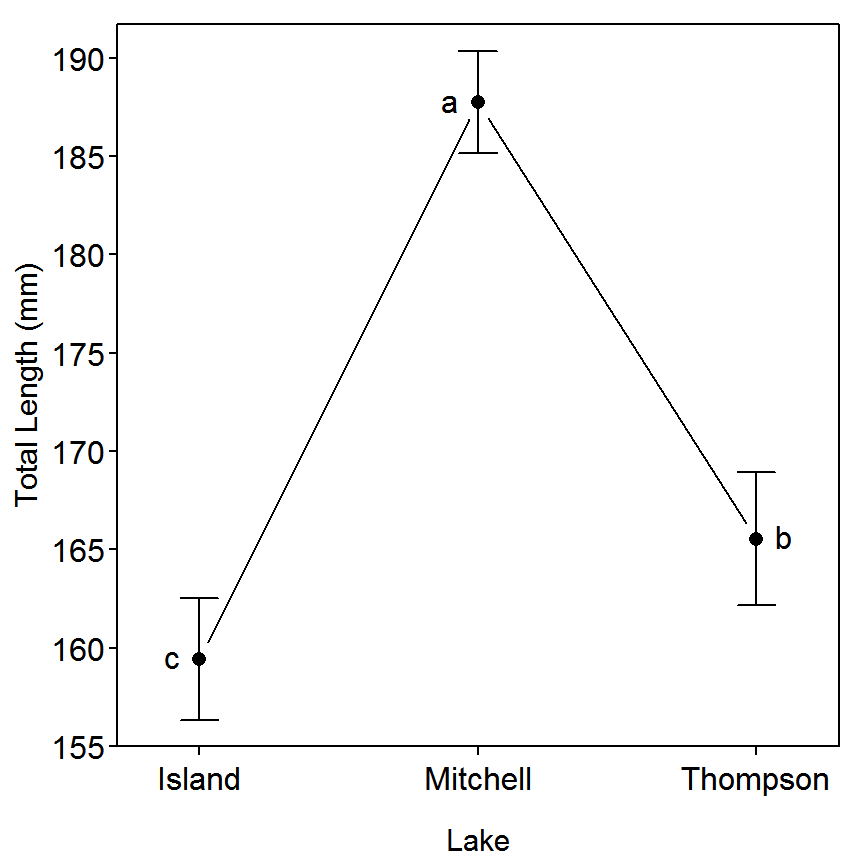### 9.1.4 Summary Table

The summary table shown at the bottom of thebox (I would have preferred doing this at the beginning) is obtained with Summarize() from the FSA package.

> Summarize(length~lake,data=d1,digits=2)
      lake   n   mean    sd min  Q1 median    Q3 max percZero
1   Island 104 159.43 15.89 122 149    160 172.2 195        0
2 Mitchell 111 187.75 13.71 145 177    192 197.5 218        0
3 Thompson 145 165.54 20.59 123 150    165 180.0 216        0

## 9.2 Testing for Differences among Length-Frequency Distributions by Means of the Kolmogorov-Smirnov Two-Sample Test

### 9.2.1 Preparing Data

The Box9_1.txt data file used here is the same file used in Box 9.1 and is not re-read here. However, as the Kolmogorov-Smirnov method described in thebox is a two-sample method. Thus, three new data frames, each of which contains only one of the lakes, must be constructed. This is most easily accomplished with Subset() (from the FSA package) which requires the original data frame as the first argument and a conditioning statement as the second argument.

> d1I <- Subset(d1,lake=="Island")     # only Island
> d1M <- Subset(d1,lake=="Mitchell")   # only Mitchell
> d1T <- Subset(d1,lake=="Thompson")   # only Thompson

### 9.2.2 Kolmogorov-Smirnov Tests

The Kolmogorov-Smirnov Test is performed in R with ks.test(). This function requires the quantitative variable from one “group” (i.e., lake) as the first argument and the quantitative variable from the second “group” as the second argument. The Komogorov-Smirnov results in the same order as presented in thebox are shown below. You will notice that R gives a warning about computing p-values because the Kolmogorov-Smirnov Test is used to compare two continuous distributions in which it would theoretically be impossible to have tied values. The discrete nature of length measurements violates this assumption.

> ks.test(d1M$length,d1T$length)
Warning in ks.test(d1M$length, d1T$length): p-value will be approximate in the
presence of ties
Two-sample Kolmogorov-Smirnov test with d1M$length and d1T$length
D = 0.5306, p-value = 8.882e-16
alternative hypothesis: two-sided 
> ks.test(d1I$length,d1T$length)
Warning in ks.test(d1I$length, d1T$length): p-value will be approximate in the
presence of ties
Two-sample Kolmogorov-Smirnov test with d1I$length and d1T$length
D = 0.1565, p-value = 0.1029
alternative hypothesis: two-sided 
> ks.test(d1I$length,d1M$length)
Warning in ks.test(d1I$length, d1M$length): p-value will be approximate in the
presence of ties
Two-sample Kolmogorov-Smirnov test with d1I$length and d1M$length
D = 0.6498, p-value < 2.2e-16
alternative hypothesis: two-sided 

### 9.2.3 Length Frequency Histograms

Given that the test above is attempting to compare the distribution of lengths among the three lakes it would be a good idea to look at these distributions. Histograms for each lake can be easily constructed with a formula in hist() as illustrated below.

> hist(length~lake,data=d1,xlab="Total Length (mm)")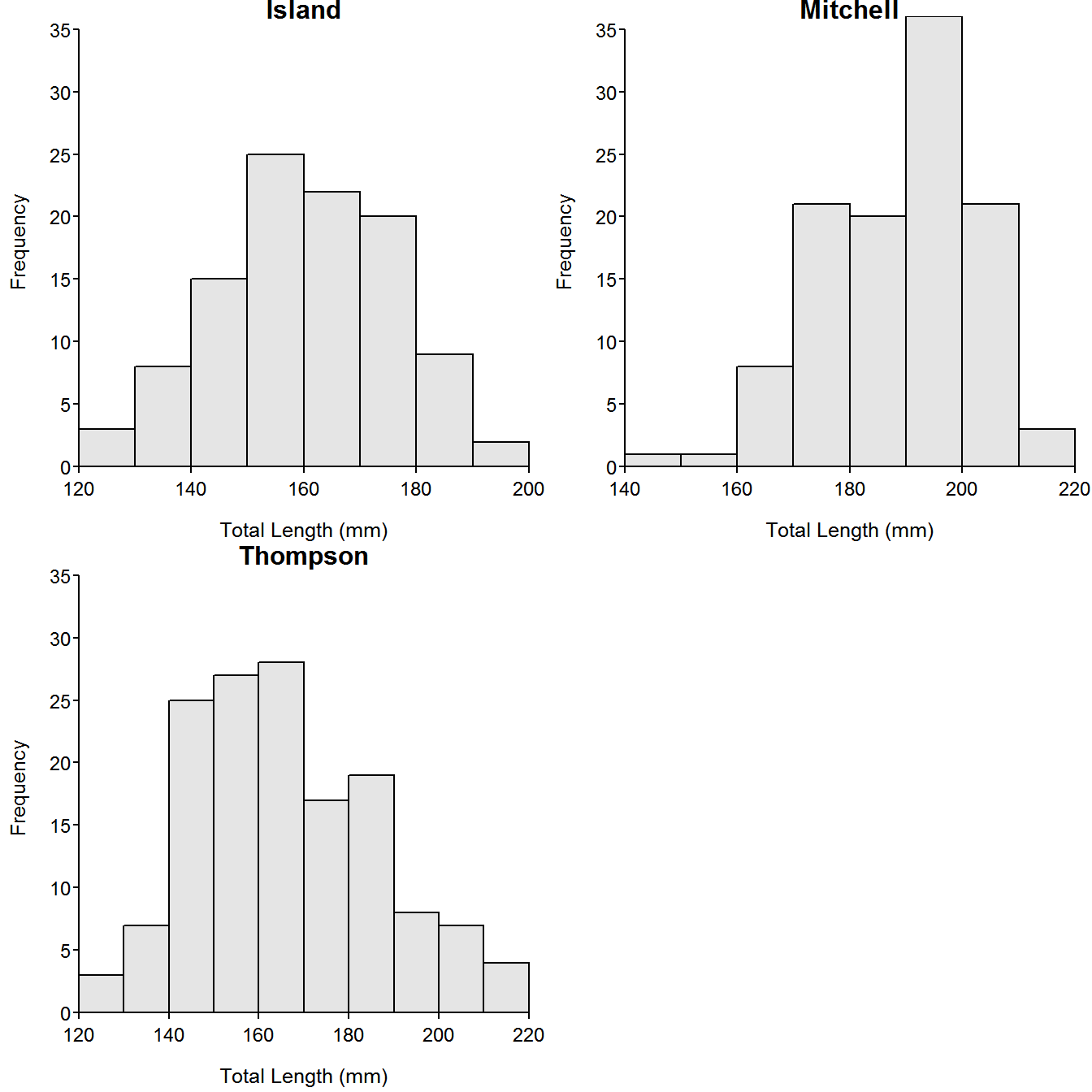Alternatively, one can look at the empirical cumulative distribution functions for each lake superimposed upon each other. The ecdf() function is used to find the empirical cumulative distribution function and add=TRUE is used to superimpose a subsequent plot on a previous plot.

> plot(ecdf(d1I$length),xlim=c(100,240),verticals=TRUE,pch=".",main="", xlab="Total Length (mm)",lwd=2) > plot(ecdf(d1M$length),col="blue",verticals=TRUE,pch=".",lwd=2,add=TRUE)
> plot(ecdf(d1T$length),col="red",verticals=TRUE,pch=".",lwd=2,add=TRUE) > legend(100,0.99,legend=c("Island","Mitchell","Thopmson"),col=c("black","blue","red"), pch=".",lwd=2,lty=1,cex=0.75)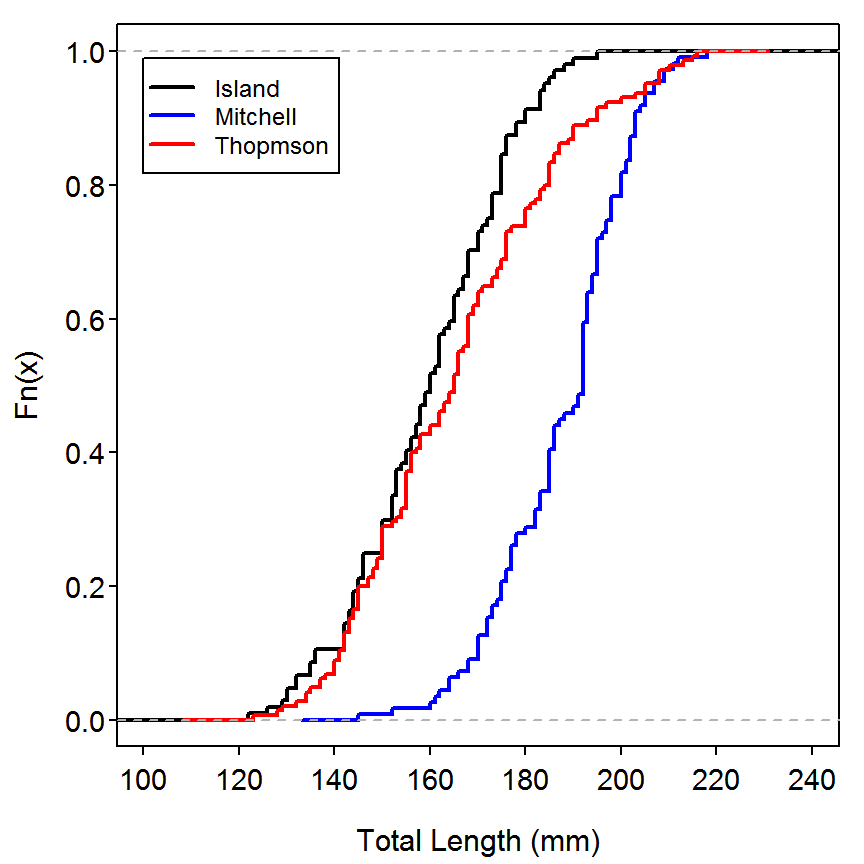## 9.3 Testing for Differences among Length-Frequency Distributions by Means of the Kruskal-Wallis Test ### 9.3.1 Preparing Data The Box9_1.txt data file used here is the same file used in Box 9.1 and is not re-read here. ### 9.3.2 Kruskal-Wallis Test The Kruskal-Wallis Test is performed in R with kruskal.test(). This function requires the response variable as the first argument and the grouping factor variable as the second argument. > kruskal.test(d1$length,d1$lake) Kruskal-Wallis rank sum test with d1$length and d1$lake Kruskal-Wallis chi-squared = 118.5671, df = 2, p-value < 2.2e-16 Histograms for each lake should be constructed as shown in Box 9.2. ## 9.4 Performing Multiple Comparisons of Length-Frequency Data As a continuation of Box 9.3, multiple comparisons following the significant Kruskal-Wallis test can be easily computed with kruskalmc(), from the pgirmess package. This function requires the response variable as the first argument and the factor variable as the second argument. > kruskalmc(d1$length,d1$lake) Multiple comparison test after Kruskal-Wallis p.value: 0.05 Comparisons obs.dif critical.dif difference Island-Mitchell 143.47375 33.99975 TRUE Island-Thompson 28.70153 32.01354 FALSE Mitchell-Thompson 114.77223 31.42022 TRUE ## 9.5 Using Contingency Tables to Test for Differences in Length-Frequency Distributions ### 9.5.1 Preparing Data As the data are presented in summarized form, it is easiest to just enter them directly into an R matrix. Doing this requires matrix() with a list of the values as the first argument, the nrow= argument to indicate the number of rows in the matrix, and the byrow=TRUE argument to tell R that the values should be placed in the matrix by rows and then by columns. The columns and rows can be named with colnames() and rownames() respectively. > d5 <- matrix(c(85,77,44,124,34,251),nrow=3,byrow=TRUE) > colnames(d5) <- c("Q","S-Q") > rownames(d5) <- c("1996","1997","1998") > d5  Q S-Q 1996 85 77 1997 44 124 1998 34 251 ### 9.5.2 Chi-Square Test I Chi-square tests are performed in R with chisq.test(). For this analysis, this function requires a matrix of the data as the only argument. The expected values and residuals ($$\frac{observed-expected}{\sqrt{expected}}$$) are obtained by appending $expected and $residuals to the saved chisq.test object. > ( chi1 <- chisq.test(d5) ) Pearson's Chi-squared test with d5 X-squared = 87.154, df = 2, p-value < 2.2e-16 > chi1$expected
            Q      S-Q
1996 42.93659 119.0634
1997 44.52683 123.4732
1998 75.53659 209.4634
> chi1$residuals  Q S-Q 1996 6.41934584 -3.85491990 1997 -0.07895125 0.04741149 1998 -4.77916601 2.86996567 The final test statistic and p-value, cell contributions to the chi-square test statistic, and a combined table of observed and expected values can also be constructed with chisq.detail(), from the TeachingDemos package. > chisq.detail(d5)  observed expected Q S-Q Total 1996 85 77 162 42.94 119.06 1997 44 124 168 44.53 123.47 1998 34 251 285 75.54 209.46 Total 163 452 615 Cell Contributions Q S-Q 1996 41.21 + 14.86 + 1997 0.01 + 0.00 + 1998 22.84 + 8.24 = 87.15 df = 2 P-value = 0  ### 9.5.3 Chi-Square Tests II The authors of the box discuss but do not show the 2x2 chi-square tests used to identify differences between pairs of years. These three tests can be constructed with chisqPostHoc(), from the NCStats package. This function requires the saved chisq.test() object as the first argument and a method to use for adjusting p-values for inflation due to multiple comparisons in the control= argument (see ?p.adjust for more discussion on the different methods for controlling the error rate with multiple comparisons). Finally, if the populations or groups to be compared were not in the rows of the original observed table (the groups in this example, i.e., the years, do form the rows so this argument is not required) then use the popsInRows=FALSE argument. The results below indicate that the PSD differs significantly among all years in the study. > chisqPostHoc(chi1,digits=6) Adjusted p-values used the fdr method.  comparison raw.p adj.p 1 1996 vs. 1997 0.000002 0.000003 2 1996 vs. 1998 0.000000 0.000000 3 1997 vs. 1998 0.000174 0.000174 ## 9.6 Testing for Differences in Size Structure by Treating Groups of Fish Caught in Each Unit of Effort as Samples ### 9.6.1 Preparing Data The Box9_6.txt data file is read and the structure is observed below. The authors create a new variable, LOGIT, that is the log of the ratio of PREF to QUAL after 0.5 had been added to each value to account for zeroes in the data. > d6 <- read.table("data/Box9_6.txt",header=TRUE) > str(d6) 'data.frame': 24 obs. of 3 variables:$ METHOD: Factor w/ 2 levels "ELEC","TOURN": 2 2 2 2 2 2 2 2 2 2 ...
$QUAL : int 3 28 13 8 61 76 38 49 62 43 ...$ PREF  : int  2 4 1 0 16 12 10 12 24 10 ...
> d6$LOGIT <- log((d6$PREF+0.5)/(d6$QUAL+0.5)) > d6  METHOD QUAL PREF LOGIT 1 TOURN 3 2 -0.3364722 2 TOURN 28 4 -1.8458267 3 TOURN 13 1 -2.1972246 4 TOURN 8 0 -2.8332133 5 TOURN 61 16 -1.3156768 6 TOURN 76 12 -1.8115621 7 TOURN 38 10 -1.2992830 8 TOURN 49 12 -1.3762440 9 TOURN 62 24 -0.9364934 10 TOURN 43 10 -1.4213857 11 TOURN 59 18 -1.1682056 12 TOURN 24 5 -1.4939250 13 ELEC 23 12 -0.6312718 14 ELEC 22 2 -2.1972246 15 ELEC 35 8 -1.4294665 16 ELEC 6 2 -0.9555114 17 ELEC 11 1 -2.0368819 18 ELEC 15 7 -0.7259370 19 ELEC 12 3 -1.2729657 20 ELEC 9 6 -0.3794896 21 ELEC 25 5 -1.5339304 22 ELEC 25 8 -1.0986123 23 ELEC 9 1 -1.8458267 24 ELEC 7 1 -1.6094379 ### 9.6.2 Summary Statistics The summary statistics of the LOGIT values for each collection type is computed with Summarize() with the first argument containing a formula of the form response~factor and the argument data= set to the data frame containing the variables (these simple statistics are different from what is presented in the box. I do not know why, as the raw data show above and the results of the linear model shown below perfectly match the results in the box). > Summarize(LOGIT~METHOD,data=d6,digits=4)  METHOD n mean sd min Q1 median Q3 max percZero 1 ELEC 12 -1.3097 0.5718 -2.197 -1.669 -1.351 -0.8981 -0.3795 0 2 TOURN 12 -1.5030 0.6294 -2.833 -1.820 -1.399 -1.2670 -0.3365 0 ### 9.6.3 Model Fitting The model fit in the box can be fit in R with lm() using the same formula and data= arguments used in Summarize() as above. The authors used a regression weighted on the number of quality fish collected. These weights are used in lm() by setting the weights= argument to QUAL. The ANOVA table is extracted from the saved lm object with anova(). > lm1 <- lm(LOGIT~METHOD,data=d6,weights=QUAL) > anova(lm1)  Df Sum Sq Mean Sq F value Pr(>F) METHOD 1 1.391 1.3908 0.2612 0.6144 Residuals 22 117.151 5.3250 Total 23 118.542  ## 9.7 Using Repeated-Measures ANOVA to Test for Size Structure Differences with Time-Dependent Data ### 9.7.1 Preparing Data The Box9_7.txt data file is read and the structure is observed below. The level names in sizegrp are observed with levels() and the site and year variables were converted to factors with factor() > d7 <- read.table("data/Box9_7.txt",header=TRUE) > str(d7) 'data.frame': 303 obs. of 4 variables:$ year   : int  1988 1988 1988 1988 1988 1988 1988 1988 1988 1988 ...
$site : int 1 1 1 2 2 2 3 3 3 4 ...$ sizegrp: Factor w/ 3 levels "age0","slot",..: 1 3 2 1 3 2 1 3 2 1 ...
$count : int 2 4 1 13 9 2 5 11 1 2 ... > levels(d7$sizegrp)
 "age0" "slot" "und" 
> d7$fsite <- factor(d7$site)
> d7$fyear <- factor(d7$year)

The authors then created a new variable, period, that indicates whether the data came from a year prior to implementation of the management regulation (i.e,. prior to 1990) or after the implementation. This variable is created by first filling the variable with “APRE” and then replacing this name with “BPOST” for all years after 1990. The new variable is then converted to a factor and the new data frame is viewed to see what was accomplished. Finally, as noted by the authors, all age-0 fish were removed from the analysis (using Subset() and noting that != means “not equals”).

> d7$period <- "APRE" # initially fill completely with "APRE" > d7$period[d7$year>1990] <- "BPOST" # then replace post-1990 with "BPOST" > d7$period <- factor(d7$period) # explicitly make a factor > view(d7)  year site sizegrp count fsite fyear period 14 1988 6 und 15 6 1988 APRE 50 1989 8 und 8 8 1989 APRE 63 1990 1 slot 3 1 1990 APRE 72 1990 4 slot 3 4 1990 APRE 235 1995 2 age0 5 2 1995 BPOST 298 1996 11 age0 0 11 1996 BPOST > d7a <- Subset(d7,sizegrp!="age0") This data frame, which has the count “stacked” for both the slot and und group must now be unstacked so that the logit of the ratio of fish in the slot to fish in the undersized category can be computed. The reshape() function is a handy, if not cumbersome, method for converting between the stacked (what reshape() calls “long”) format to unstacked (what reshape() calls “wide”) format. For our purposes, reshape() requires four arguments: • data: the data frame to be converted from (note that this is the first argument) • direction: this is the format to be converted to (i.e., we are converting from “long” to “wide”) • timevar: this is the variable that contains the information on how the “long” data should be split into “wide” data. In this case, we want to have count separated into two columns, one for undersized fish and one for slot length fish. • idvar: this is the variable or variables that will are repeated in the “long” format and should occur only once in the “wide” format. Thus, the appropriate reshape() command for this example is shown below with the resulting data frame viewed. Notice that the counts of under- and slot-sized fish are contained in the count.und and count.slot variables for each year, site, and period combination. > d7b <- reshape(d7a,direction="wide",timevar="sizegrp",idvar=c("site","year","fyear","period")) > view(d7b)  year site fyear period count.und fsite.und count.slot fsite.slot 19 1989 1 1989 APRE 7 1 1 1 35 1989 9 1989 APRE 10 9 3 9 91 1992 3 1992 BPOST 8 3 1 3 111 1993 2 1993 BPOST 8 2 4 2 147 1994 8 1994 BPOST 2 8 1 8 189 1996 6 1996 BPOST 3 6 3 6 Finally, the undert, slott, total, and LOGIT variables, as described in the box, are constructed. > d7b$undert <- d7b$count.und+0.5 > d7b$slott <- d7b$count.slot+0.5 > d7b$total <- d7b$undert + d7b$slott
> d7b$LOGIT <- log(d7b$slott/d7b$undert) > head(d7b) # first 6 rows  year site fyear period count.und fsite.und count.slot fsite.slot undert 1 1988 1 1988 APRE 4 1 1 1 4.5 3 1988 2 1988 APRE 9 2 2 2 9.5 5 1988 3 1988 APRE 11 3 1 3 11.5 7 1988 4 1988 APRE 8 4 7 4 8.5 9 1988 6 1988 APRE 15 6 2 6 15.5 11 1988 7 1988 APRE 10 7 0 7 10.5 slott total LOGIT 1 1.5 6 -1.0986123 3 2.5 12 -1.3350011 5 1.5 13 -2.0368819 7 7.5 16 -0.1251631 9 2.5 18 -1.8245493 11 0.5 11 -3.0445224 ### 9.7.2 Normality Tests A variety of normality tests are available in R (see Box 3.9 in the Chapter 3 vignette). The Shapiro-Wilks test (the authors of the box refer to a “Wilk’s Lambda” test of normality. I do not believe that this is the correct term; Wilk’s Lambda is used in multivariate means testing – see this short online article and assume that they mean Shapiro-Wilks normality test) is conducted with shapiro.test(). The only required argument is a vector on which to test normality. > shapiro.test(d7b$LOGIT[d7b$period=="APRE"]) Shapiro-Wilk normality test with d7b$LOGIT[d7b$period == "APRE"] W = 0.9579, p-value = 0.2402 > shapiro.test(d7b$LOGIT[d7b$period=="BPOST"]) Shapiro-Wilk normality test with d7b$LOGIT[d7b$period == "BPOST"] W = 0.9734, p-value = 0.1486 The two normal quantile plots (presumably produced in the SAS program shown in the box) are constructed with qqnorm(). > qqnorm(d7b$LOGIT[d7b$period=="APRE"],main="Pre-Regulation")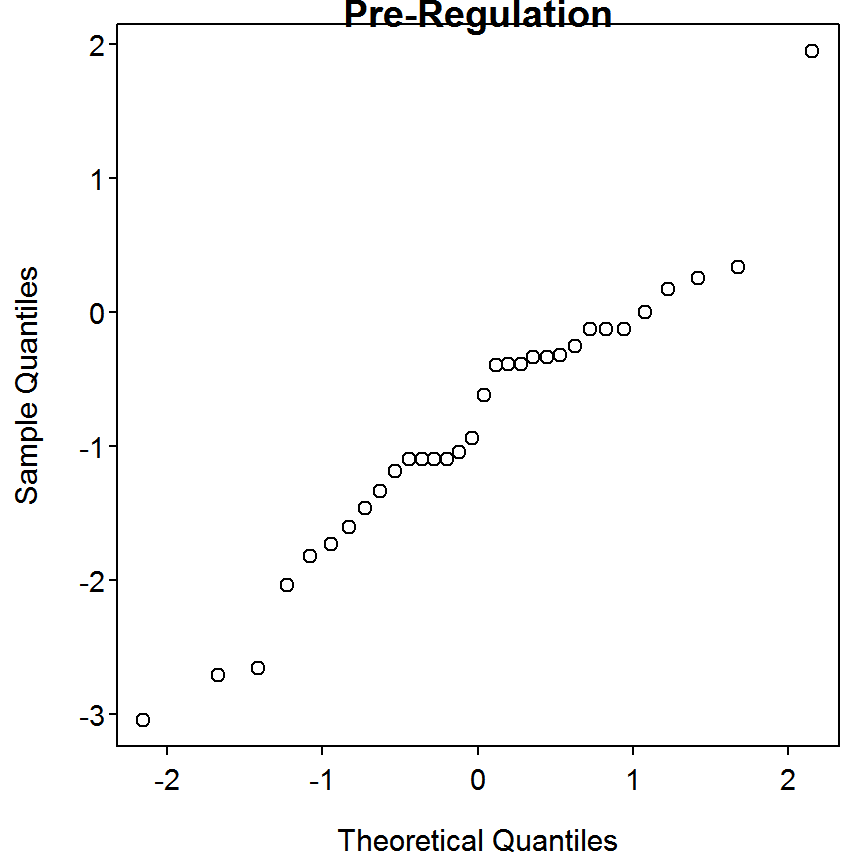> qqnorm(d7b$LOGIT[d7b\$period=="BPOST"],main="Post-Regulation")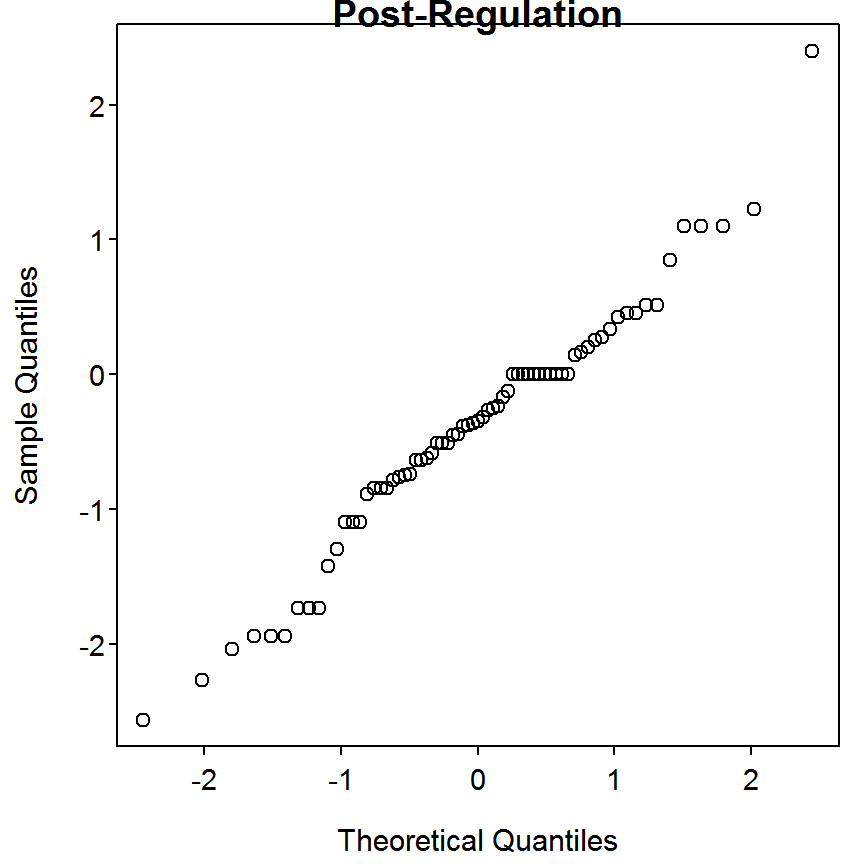### 9.7.3 Repeated Measures ANOVA

THIS SECTION HAS NOT YET BEEN CONVERTED

Reproducibility Information
Compiled Date: Thu May 14 2015
Compiled Time: 2:41:55 PM
Code Execution Time: 6.86 s

R Version: R version 3.2.0 (2015-04-16)
System: Windows, i386-w64-mingw32/i386 (32-bit)
Base Packages: base, datasets, graphics, grDevices, methods, stats, utils
Required Packages: FSA, NCStats, lattice, multcomp, nlme, pgirmess,
TeachingDemos and their dependencies (boot, car, codetools, dplyr,
FSAdata, gdata, gplots, graphics, grDevices, grid, Hmisc, knitr,
lmtest, mvtnorm, plotrix, relax, rgdal, sandwich, sciplot, sp, spdep,
splancs, stats, survival, TH.data, utils)
Other Packages: car_2.0-25, FSA_0.6.13, FSAdata_0.1.9, knitr_1.10.5,
lattice_0.20-31, multcomp_1.4-0, mvtnorm_1.0-2, NCStats_0.4.3,
nlme_3.1-120, pgirmess_1.6.0, rmarkdown_0.6.1, survival_2.38-1,
TeachingDemos_2.9, TH.data_1.0-6
boot_1.3-16, caTools_1.17.1, cluster_2.0.1, coda_0.17-1,
codetools_0.2-11, colorspace_1.2-6, DBI_0.3.1, deldir_0.1-9,
digest_0.6.8, dplyr_0.4.1, evaluate_0.7, foreign_0.8-63, formatR_1.2,
Formula_1.2-1, gdata_2.16.1, ggplot2_1.0.1, gplots_2.17.0, grid_3.2.0,
gridExtra_0.9.1, gtable_0.1.2, gtools_3.4.2, highr_0.5, Hmisc_3.16-0,
htmltools_0.2.6, KernSmooth_2.23-14, latticeExtra_0.6-26,
LearnBayes_2.15, lme4_1.1-7, lmtest_0.9-33, magrittr_1.5, MASS_7.3-40,
Matrix_1.2-0, mgcv_1.8-6, minqa_1.2.4, munsell_0.4.2, nloptr_1.0.4,
nnet_7.3-9, parallel_3.2.0, pbkrtest_0.4-2, plotrix_3.5-11, plyr_1.8.2,
proto_0.3-10, quantreg_5.11, RColorBrewer_1.1-2, Rcpp_0.11.6,
relax_1.3.15, reshape2_1.4.1, rgdal_0.9-2, rpart_4.1-9, sandwich_2.3-3,
scales_0.2.4, sciplot_1.1-0, sp_1.1-0, SparseM_1.6, spdep_0.5-88,
splancs_2.01-37, splines_3.2.0, stringi_0.4-1, stringr_1.0.0,
tools_3.2.0, yaml_2.1.13, zoo_1.7-12